Clutch Prep is now a part of Pearson
Ch.7 - Quantum MechanicsWorksheetSee all chapters

# Electromagnetic Spectrum

See all sections
Sections
Wavelength and Frequency
Speed of Light
The Energy of Light
Electromagnetic Spectrum
Photoelectric Effect
De Broglie Wavelength
Heisenberg Uncertainty Principle
Bohr Model
Emission Spectrum
Bohr Equation
Introduction to Quantum Mechanics
Quantum Numbers: Principal Quantum Number
Quantum Numbers: Angular Momentum Quantum Number
Quantum Numbers: Magnetic Quantum Number
Quantum Numbers: Spin Quantum Number
Quantum Numbers: Number of Electrons
Quantum Numbers: NodesJules Bruno

# Electromagnetic spectrum

## What is the electromagnetic spectrum

Before us we have the electromagnetic spectrum. The electromagnetic spectrum is the different forms of radioactive energy that exists in our universe. The small portion that we can see with our naked eye is the visible light spectrum. Something like this here will not be given on your exams so it is important that you remember the order of the electromagnetic spectrum.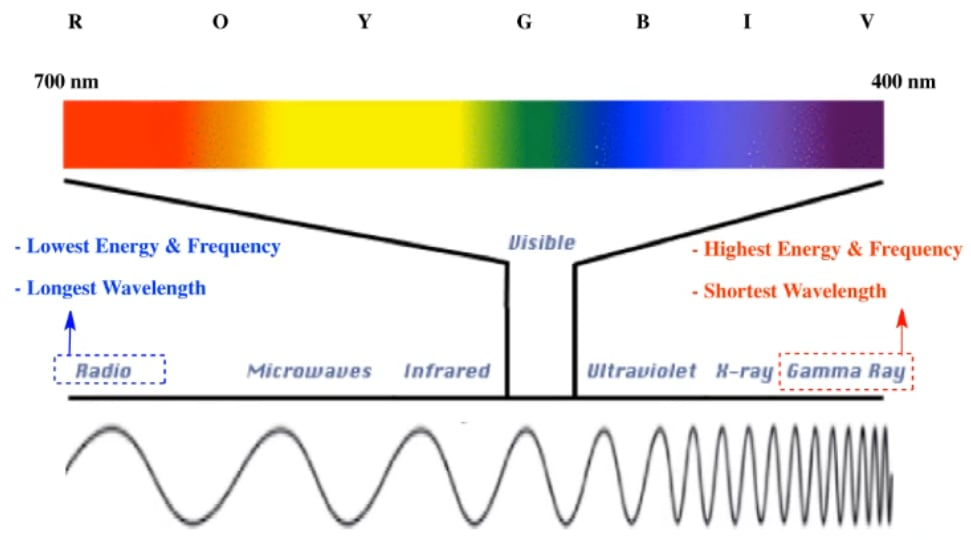If we take a look here, we should realize that the electromagnetic spectrum tries to relate three variables to one another

1. Energy

2. Frequency

3. Wavelength.

## Electromagnetic spectrum frequency

Frequency is represented by the letter mu. Frequency is how many waves we get within a given amount of time. The waves that we’re referring to are these waves down here.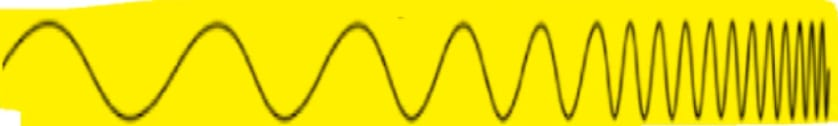Let’s say that this to this represented one second. Within that once second, we can see that there’s not that many waves within that given amount of time, so the frequency will be very low.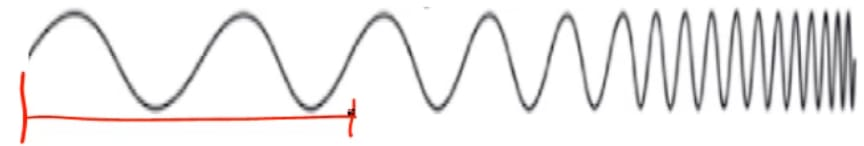But as we move more to the right, we can see that there’s and more packing of waves within about the same amount of time. That means our frequency is increasing. We’re getting more waves per given amount of time.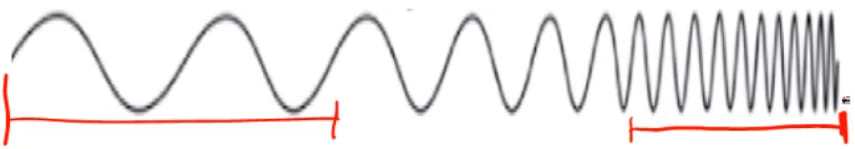## Electromagnetic spectrum wavelength

We’re going to say here that wavelength, which is represented by the symbol lambda,  represents the distance from one crest of the wave, which is the top, to the next. We can see here that the wavelength, the distance between one wave to the next, is very large. But as we go more to the right, the distance between the waves is decreasing.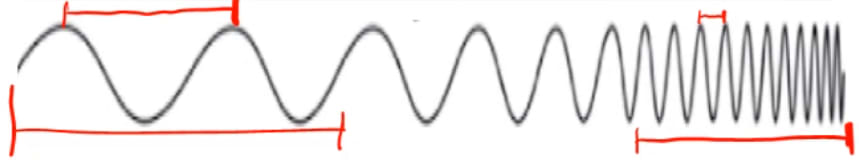This is important because as we can see, there is some type of relationship we can establish. We can see that as we’re going from left to right, the wavelength gets shorter. It was the longest on this end but then it shortens as it gets over to this end.

## Electromagnetic spectrum energy

The opposite is happening to frequency and energy. On this end, the frequency and energy were very low, but then as we go more to the right, they get higher. We’re going to say here that energy and frequency are directly related. That means that they increase together or decrease together. Besides directly related, you may hear that they are directly proportional to one another. If one increases, the other one increases. They are the opposite of wavelength. Opposite, another term for it we could say is inversely proportional. That means if they’re increasing, that means wavelength is decreasing. Wavelength is inversely proportional or the opposite of energy and frequency.

## Electromagnetic spectrum visible light

Again, you’re not going to be given this chart so you have to remember the order. Radio waves have the longest wavelength, and therefore the lowest frequency and energy. Next we’d have microwaves, then infrared. The visible light spectrum is the part that we can see. Remember, with the visible light spectrum we have ROYGBIV which stands for red light, orange light, yellow light, green light, blue light, indigo and violet. After visible, we have ultraviolet, then we have x-ray and then finally, gamma rays which have the shortest wavelength and therefore the highest energy and frequency.

When it comes to ROYGBIV, if you can't recall what side ROY is on and what side BIV is on, infrared has red in it that’s why it’s next to the red portion. Ultraviolet has violet in it that’s why it’s next to the violet portion. It also follows the same trend. As we move from left to right, wavelength is decreasing.

Here, we’re going to say the visible light spectrum is typically between 700 nanometers and 400 nanometers in terms of its wavelength. Nanometers is referring to the wavelength of these different colors of light. Again, you won't be given this electromagnetic spectrum. It’s essential that you remember the relationship it shares with these 3 variables and then the order that it’s presented.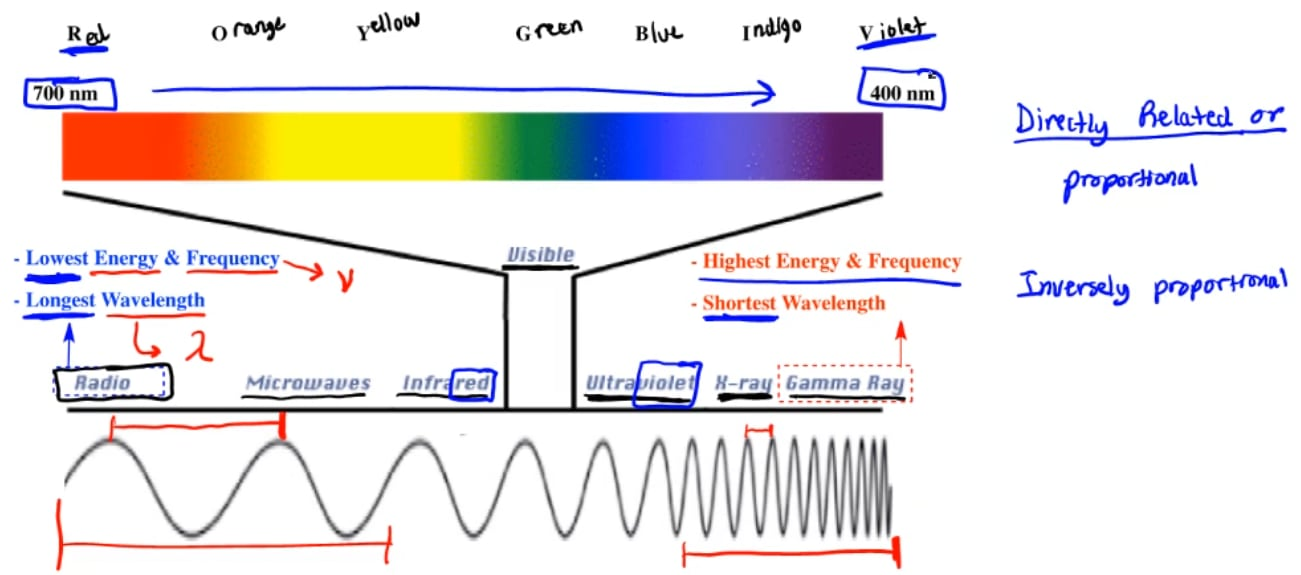Jules Bruno

Jules felt a void in his life after his English degree from Duke, so he started tutoring in 2007 and got a B.S. in Chemistry from FIU. He’s exceptionally skilled at making concepts dead simple and helping students in covalent bonds of knowledge.